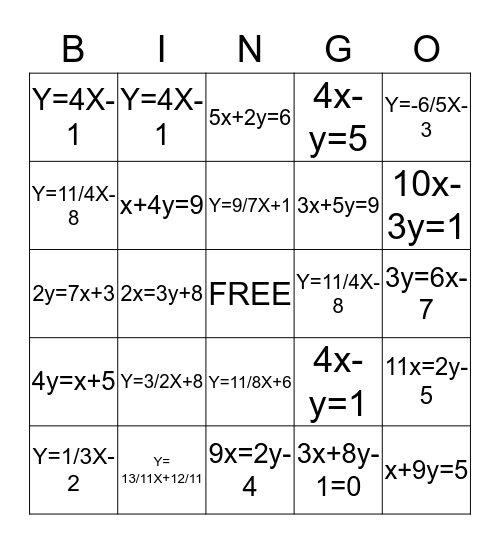# Slope-Intercept Form BingoThis bingo card has a free space and 24 words: Y=3/2X+8, Y= 13/11X+12/11, Y=9/7X+1, Y=1/3X-2, Y=-6/5X-3, Y=4X-1, Y=11/4X-8, Y=4X-1, Y=11/4X-8, Y=11/8X+6, 3x+5y=9, 2y=7x+3, 4x-y=1, 11x=2y-5, x+4y=9, 3x+8y-1=0, 3y=6x-7, 5x+2y=6, 10x-3y=1, 2x=3y+8, x+9y=5, 4y=x+5, 4x-y=5 and 9x=2y-4.

⚠ This card has duplicate items: Y=4X-1 (2), Y=11/4X-8 (2)

## Play Online